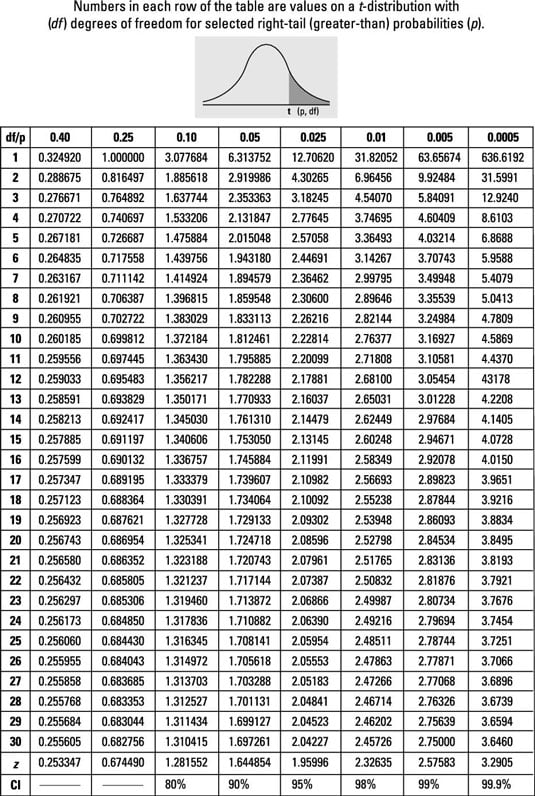PROBABILITY AND STATISTICS

# 🆕 T-Table To Solve Statistics Problems

Method Of Finding Value from T-Table

1. For a study involving one population and a sample size of 18 (assuming you have a t-distribution), what row of the t-table will you use to find the right-tail (“greater than”) probability affiliated with the study results?

The study involving one population and a sample size of 18 has n – 1 = 18 – 1 = 17 degrees of freedom.

2. For a study involving a paired design with a total of 44 observations, with the results assuming a t-distribution, what row of the table will you use to find the probability affiliated with the study results?

A matched-pairs design with 44 total observations has 22 pairs. The degrees of freedom is one less than the number of pairs: n – 1 = 22 – 1 = 21.

3. t-value of 2.35, from a t-distribution with 14 degrees of freedom, has an upper-tail (“greater than”) probability between which two values on the t-table?

Using the t-table, locate the row with 14 degrees of freedom and look for 2.35. However, this exact value doesn’t lie in this row, so look for the values on either side of it: 2.14479 and 2.62449. The upper-tail probabilities appear in the column headings; the column heading for 2.14479 is 0.025, and the column heading for 2.62449 is 0.01.

Hence, the upper-tail probability for a t-value of 2.35 must lie between 0.025 and 0.01.### Ashok Nath

Ashok Nath is an Author in Surajchand99.com.np and is pursuing Bachelor's Degree In Computer Engineering From Nepal College Of Information Technology (NCIT) Pokhara University Nepal.